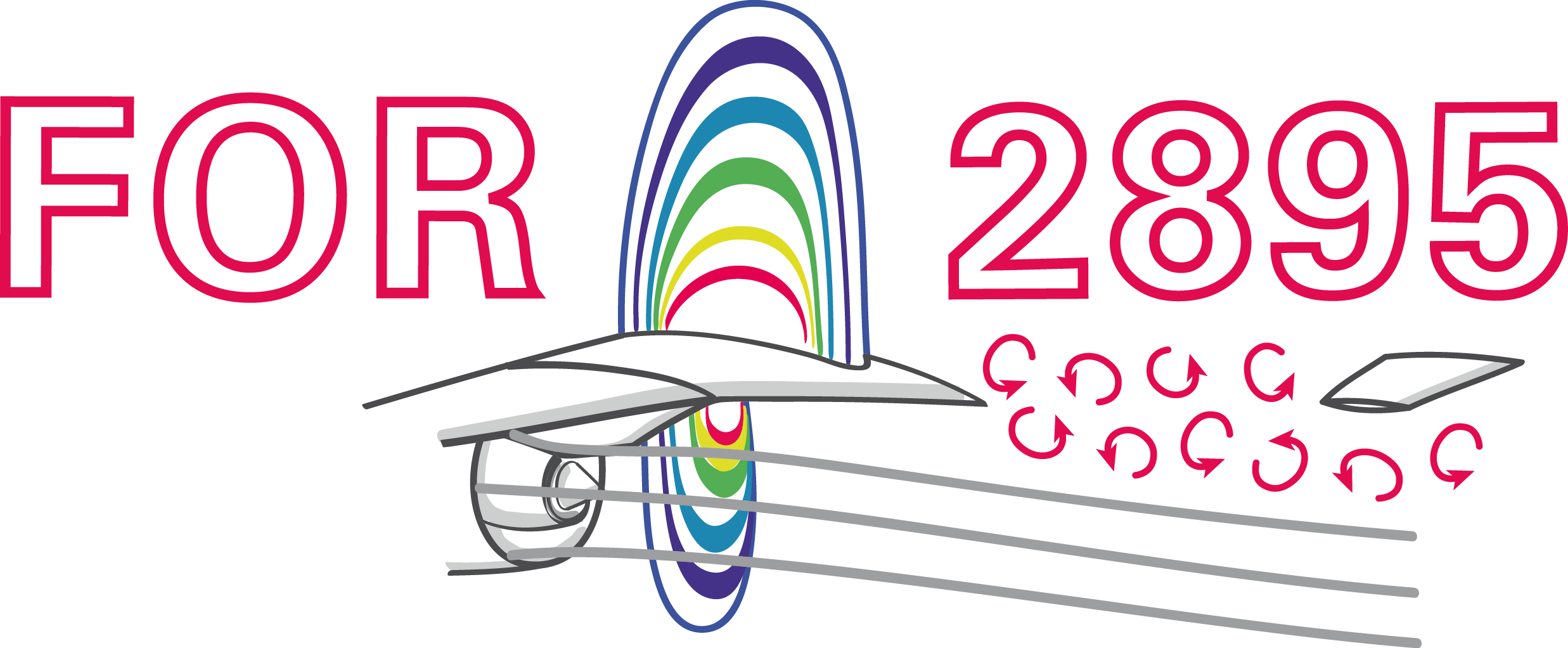### Model-based deep reinforcement learning for flow control

Andre Weiner, Janis Geise
TU Braunschweig, Institute of Fluid Mechanics

## Outline

1. Closed-loop active flow control
2. Proximal policy optimization (PPO)
3. Model-based PPO

## Closed-loop active flow control

Goals of flow control:

• drag reduction
• process intensification
• noise reduction
• ...

Categories of flow control:

1. passive: geometry, fluid properties, ...
2. active: blowing/suction, heating/cooling, ...

energy input vs. efficiency gain

Categories of active flow control:

1. open-loop: actuation predefined
2. closed-loop: actuation based on sensor input

How to find the control law?

Closed-loop flow control with variable Reynolds number; source: F. Gabriel 2021.

Why CFD-based DRL?

• save virtual environment
• prior optimization, e.g., sensor placement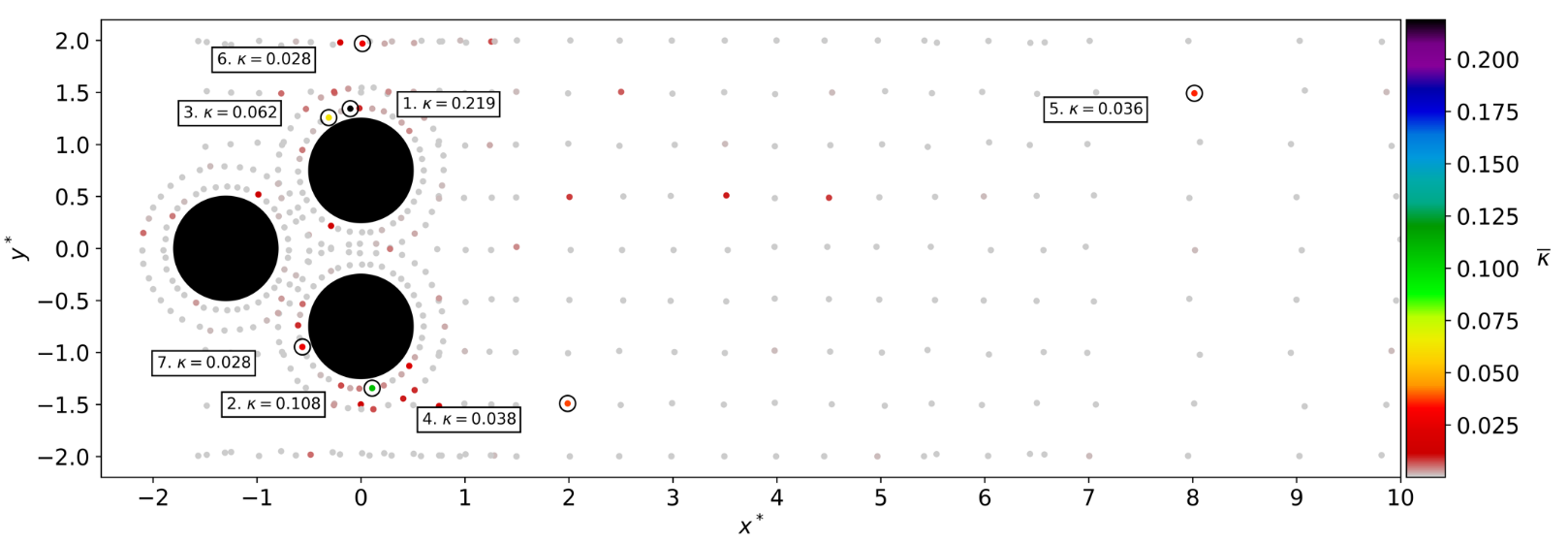Time-averaged attention weights $\bar{\kappa}$
Tom Krogmann 10.5281/zenodo.7636959

Training cost DrivAer model

• $8$ hours/simulation (2000 MPI ranks)
• $10$ parallel simulations
• $3$ episodes/day
• $60$ days/training (180 episodes)
• $60\times 24\times 10\times 2000 \approx 30\times 10^6$ core hours

CFD environments are expensive!

## Proximal policy optimizationCreate an intelligent agent that learns to map states to actions such that expected returns are maximized.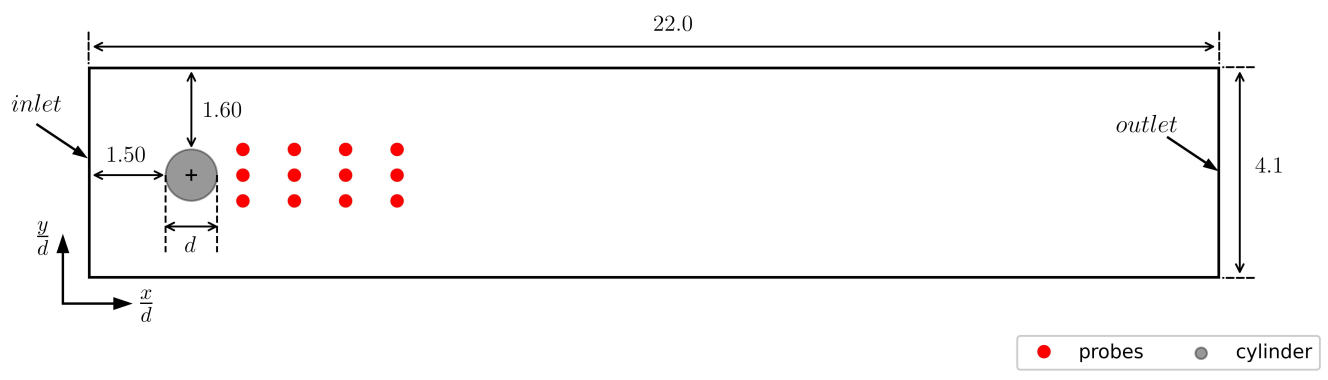Flow past a cylinder benchmark.

What is our goal?

$r=3-(c_d + 0.1 |c_l|)$

$c_d$, $c_l$ - drag and lift coeff.; see J. Rabault et al.

Long-term consequences:

$$G_t = \sum\limits_{l=0}^{N_t-t} \gamma^l R_{t+l}$$

• $t$ - control time step
• $G_t$ - discounted return
• $\gamma$ - discount factor, typically $\gamma=0.99$
• $N_t$ - number of control steps

What to expect in a given state?

$$L_V = \frac{1}{N_\tau N_t} \sum\limits_{\tau = 1}^{N_\tau}\sum\limits_{t = 1}^{N_t} \left( V(s_t^\tau) - G_t^\tau \right)^2$$

• $\tau$ - trajectory (single simulation)
• $s_t$ - state/observation (pressure)
• $V$ - parametrized value function
• clipping not included

Was the selected action a good one?

$$\delta_t = R_t + \gamma V(s_{t+1}) - V(s_t)$$ $$A_t^{GAE} = \sum\limits_{l=0}^{N_t-t} (\gamma \lambda)^l \delta_{t+l}$$

• $\delta_t$ - one-step advantage estimate
• $A_t^{GAE}$ - generalized advantage estimate
• $\lambda$ - smoothing parameter

Making good actions more likely:

$$J_\pi = \frac{1}{N_\tau N_t} \sum\limits_{\tau = 1}^{N_\tau}\sum\limits_{t = 1}^{N_t} \left( \frac{\pi(a_t|s_t)}{\pi^{old}(a_t|s_t)} A^{GAE,\tau}_t\right)$$

• $\pi$ - current policy
• $\pi^{old}$ - old policy (previous episode)
• clipping and entropy not included
• $J_\pi$ is maximized

## Model-based PPO

Janis Geise, Github, 10.5281/zenodo.7642927

Idea: replace CFD with model(s) in some episodes


for e in episodes:
if models_reliable():
sample_trajectories_from_models()
else:
sample_trajectories_from_simulation()
update_models()
update_policy()


Based on Model Ensemble TRPO.

When are the models reliable?

1. evaluate policy for every model
2. compare to previous policy loss
3. switch if loss did not decrease for
at least $50\%$ of the models

How to sample from the ensemble?

1. pick initial sequence from CFD
2. fill buffer
1. select random model
2. sample action
3. predict next state

Recipe to create env. models:

• input/output normalization
• fully-connected, feed-forward
• time delays (~30)
• layer normalization
• batch training (size ~100)
• learning rate decay (on plateau)
• "early stopping"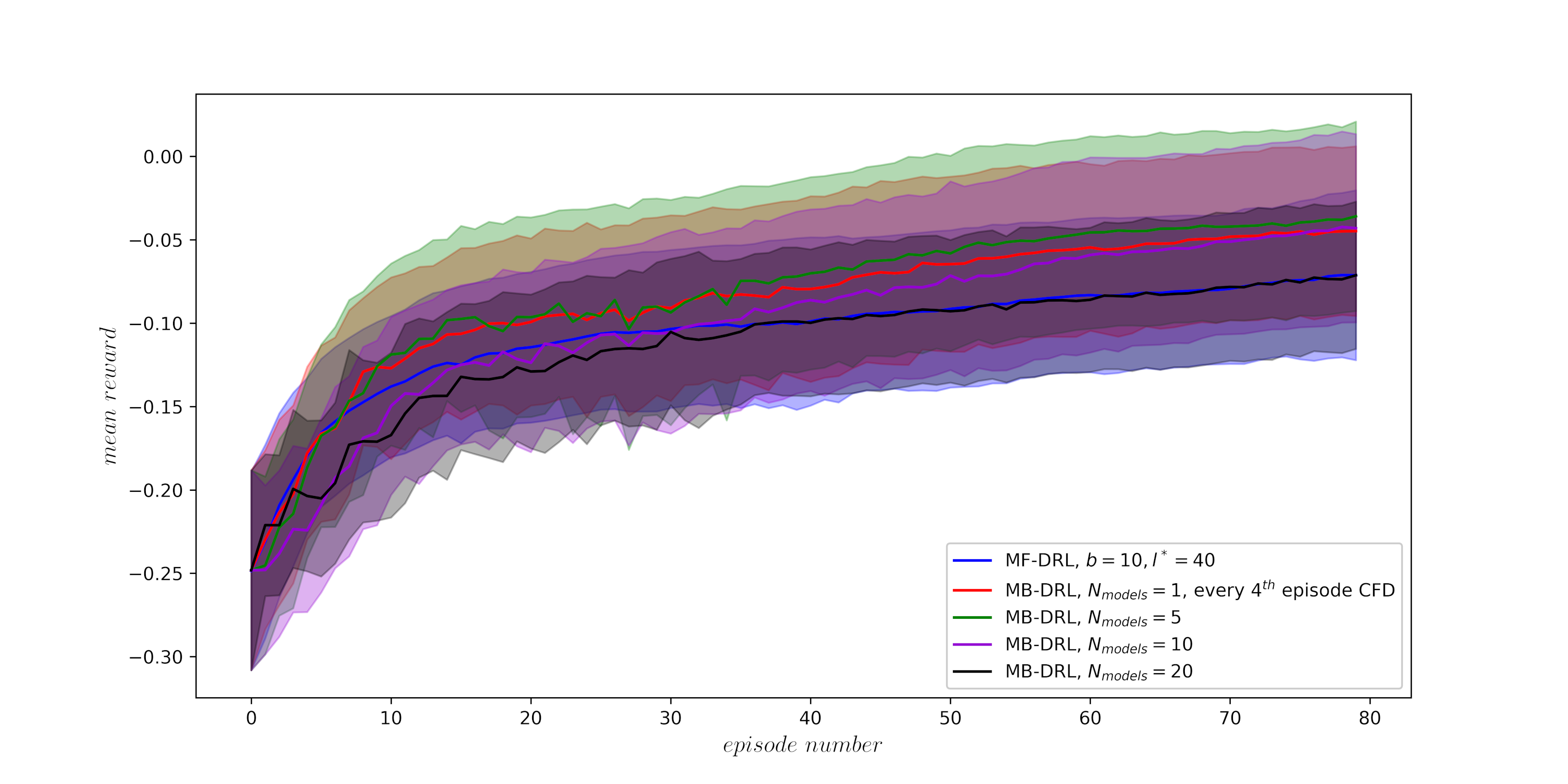Influence on the number of models; average over trajectories and 3 seeds.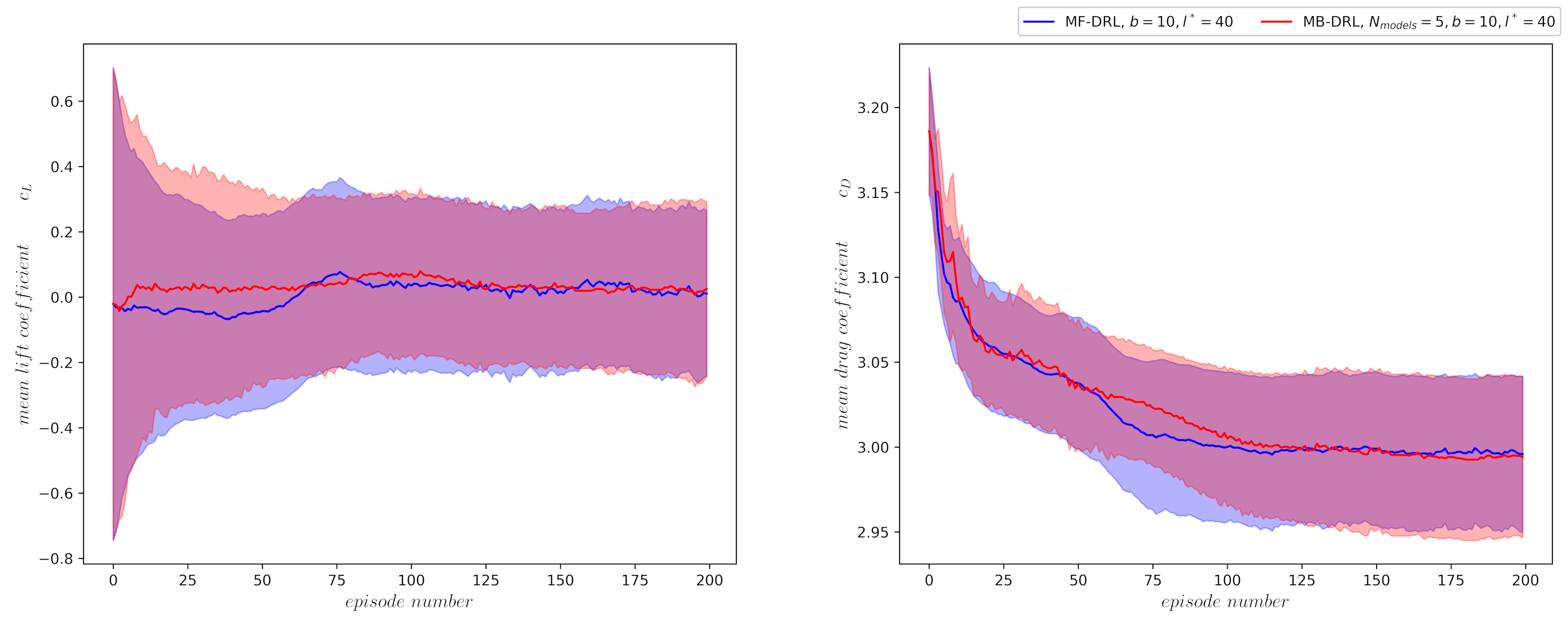Comparison of best policy over 3 seeds.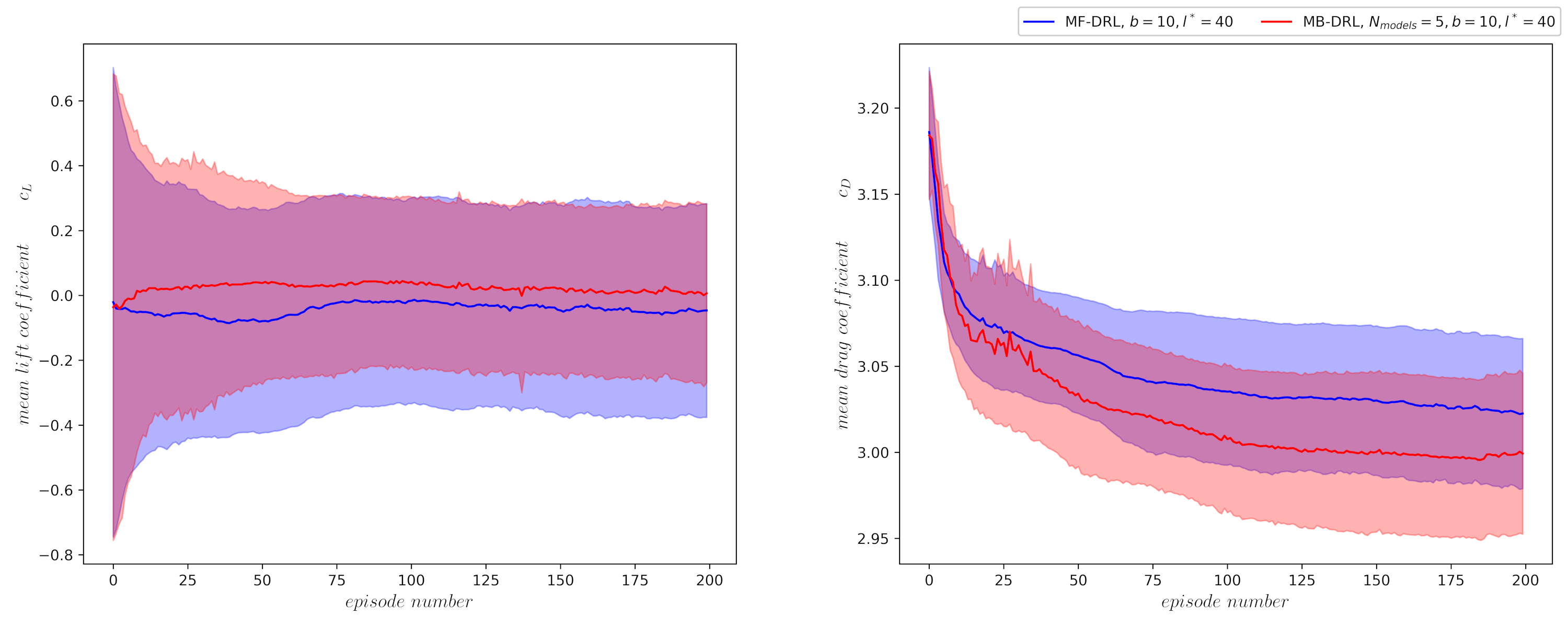Average performance over 3 seeds.

What are the savings?

$50-70\%$ in training time

# THE END

### Thank you for you attention!